Basic Mathematics

# Geometric Manipulation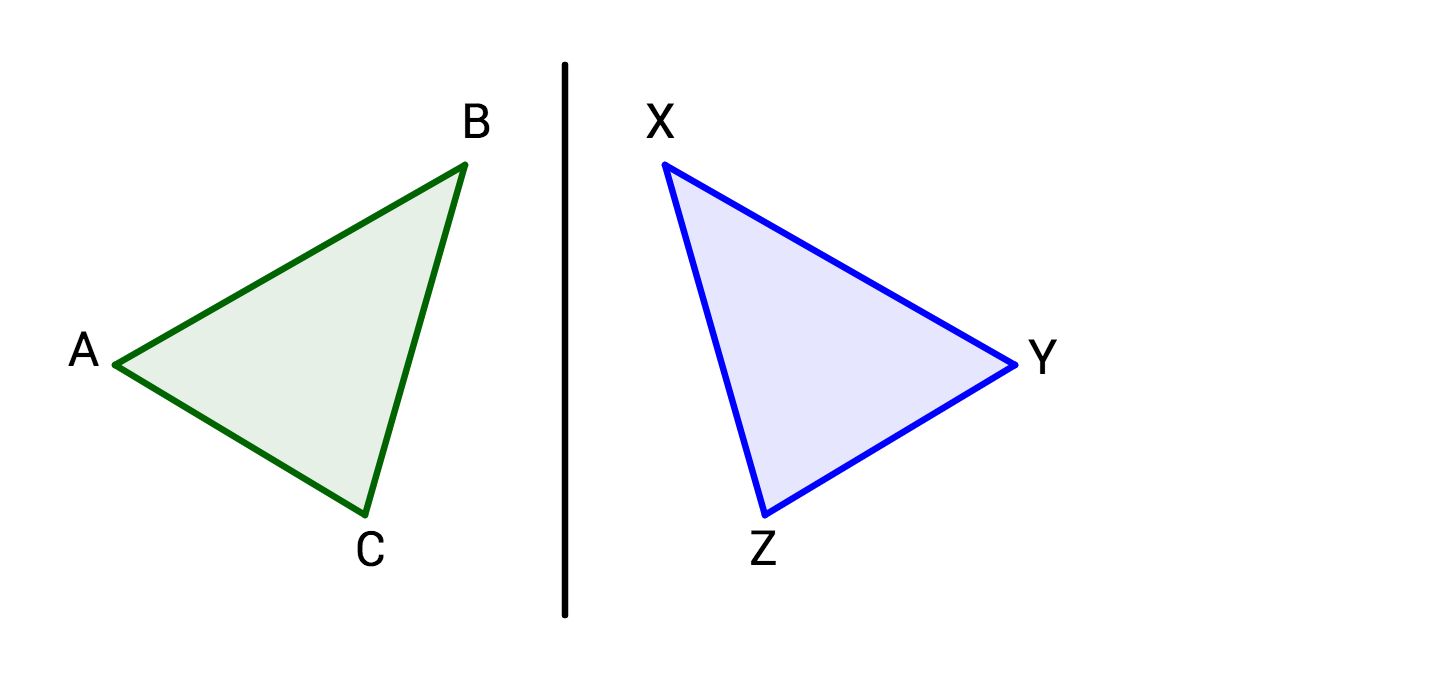The green triangle is reflected over the line to create the blue triangle. Angle $A$ in the green triangle has the same measure as which angle in the blue triangle?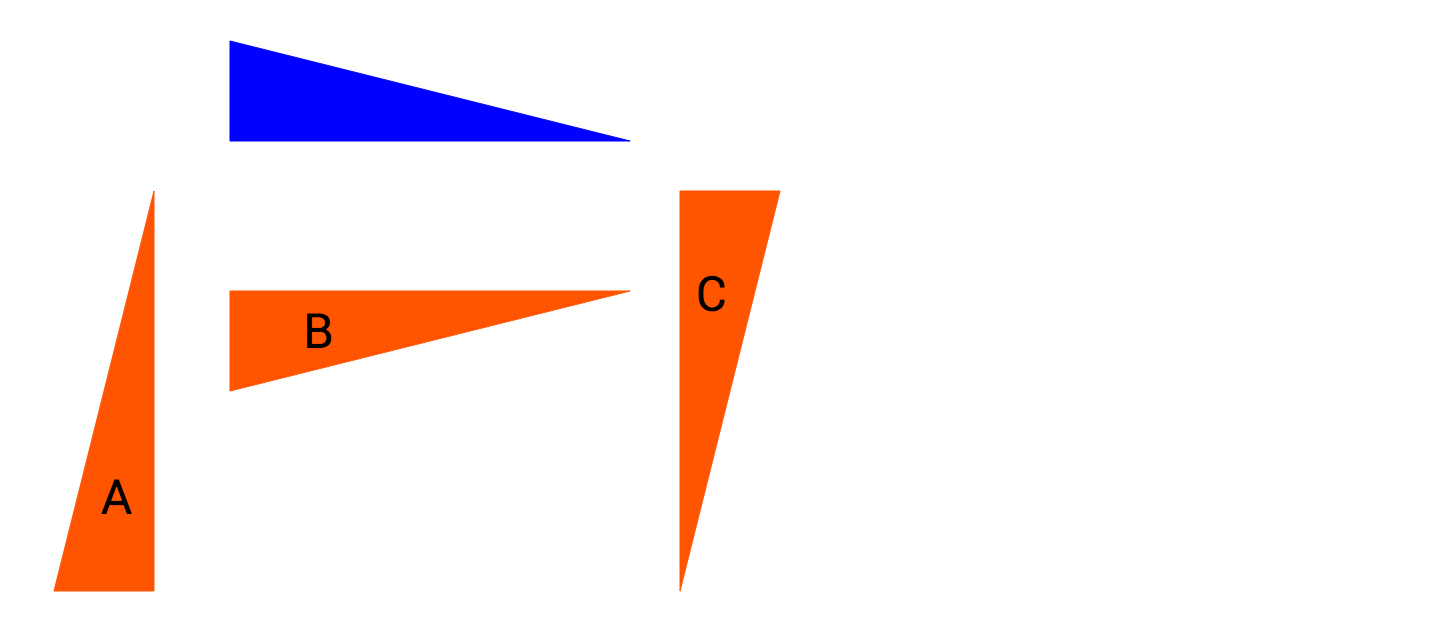Which triangle CANNOT be rotated to create the blue triangle?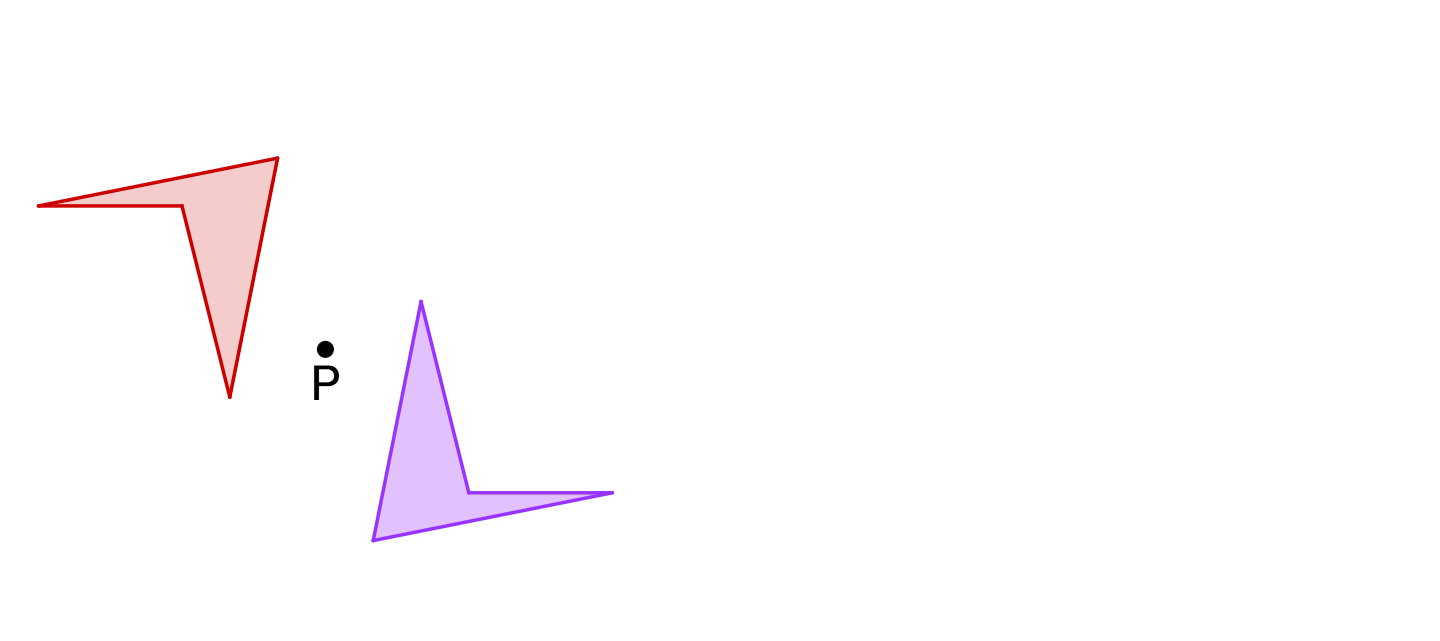If the red figure is rotated around point $P$ to become the purple figure, how many degrees does it need to rotate?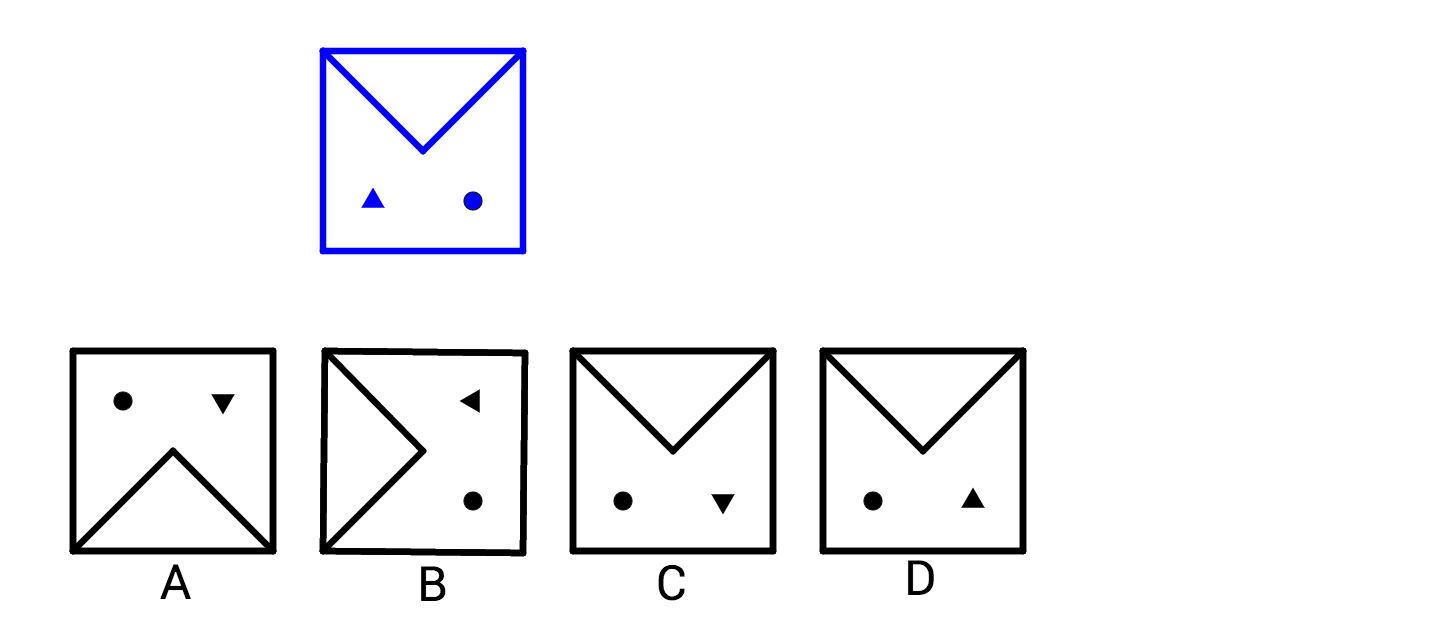Which image is a vertical or horizontal reflection (and only a vertical or horizontal reflection) of the blue design?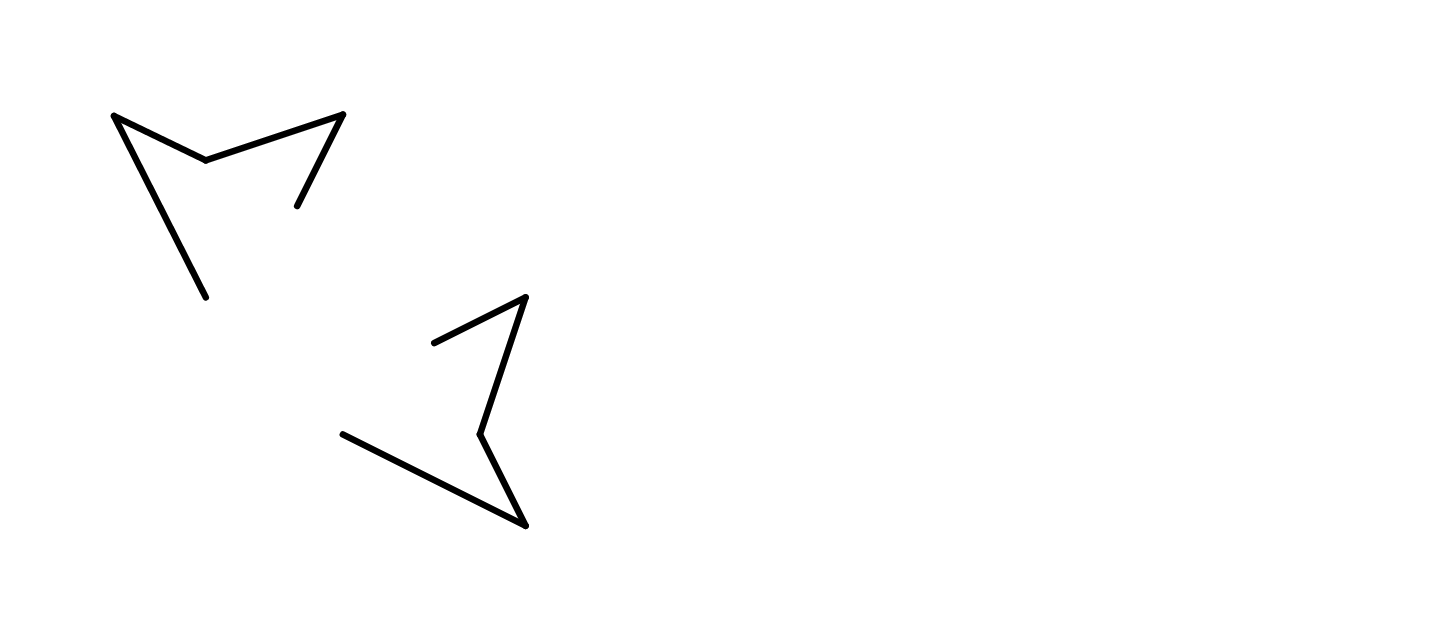Do the two figures have a vertical, horizontal, or diagonal line of symmetry?

×